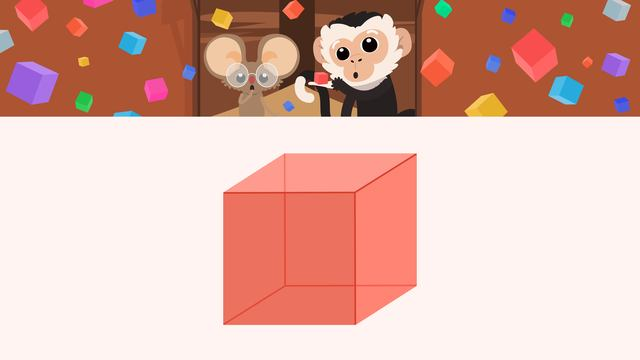# CubesRating

Ø 5.0 / 1 ratings

The authorsTeam Digital
Cubes
CCSS.MATH.CONTENT.2.G.A.1

## Basics on the topicCubes

### In This Video

Niko and Nia see a shape storm that rains cubes. Except, Niko doesn’t know what it is. So he wonders ‘what is a cube in math?’ Join in to learn all about cubes!

### Cube (Math) Explanation & Example

You may wonder ‘what is a cube in math’, or ‘what does cube mean in math’. Well, a cube in math is a 3-D shape that has specific defining attributes, or characteristics.

The defining attributes of a cube are:

• Six square faces
• Eight vertices, or corners
• Twelve edges, or sidesTherefore, the cube definition (math) is a 3-D shape that has six square faces, eight vertices, and twelve edges. This can also be referred to as the cube rule (math).

Underneath, you will find a math cube worksheet, where you can practice identifying if a shape is a cube or not based on the attributes you learned.

### TranscriptCubes

"Hey Nia, do you hear that?" Uh oh, it looks like there is a shape storm happening! "Woooaaaaah. This is new!" "I wonder what this shape is?" Let's join Nico and Nia as they learn all about cubes. A cube is a 3-D shape. A 3-D shape is a solid figure that has three dimensions; length, width, and height. 3-D shapes can be identified by defining attributes, or properties, that will not change, and non-defining attributes, or properties, which may change. Let's take a look at defining attributes of a cube. A cube has six faces, which are made up of squares. A cube also has eight vertices, or corners! Finally, a cube has twelve edges, which is where two faces meet. A shape must have all three of these defining attributes to be classified as a cube. Now let's look at non-defining attributes of cubes, or things that may change. A cube may be different colors. A cube may be different sizes. A cube may have different orientations, or be turned differently. To identify cubes, we look for the defining attributes, or properties, that do change. Let's practice identifying cubes! Is this a cube? First, count the square faces. There are six square faces, it might be a cube! Now count the vertices. There are eight vertices, it might still be a cube! Finally, count the edges. There are twelve edges. Since all the defining properties of a cube are present, this is a cube! Let's look at this shape. Is this a cube? Start by counting the faces. There are five faces, and none of them are square. Since a cube must have six square faces, this is not a cube! Is this a cube? This is a cube, because there are six square faces, eight vertices, and twelve edges! Even though the two cubes we saw are different colors, different sizes, and have different orientations from each other they are still cubes because the defining attributes are present! Before we see what Nico and Nia are up to in the shape storm, let's review! Remember, a cube may have non-defining attributes such as, a different color, a different size, or a different orientation! In order to be classified as a cube, it must have all of the following defining attributes: Six square faces, eight vertices, and twelve edges! "Huh?" "We should close the window!" "Yes Nico, that is probably a good idea!"